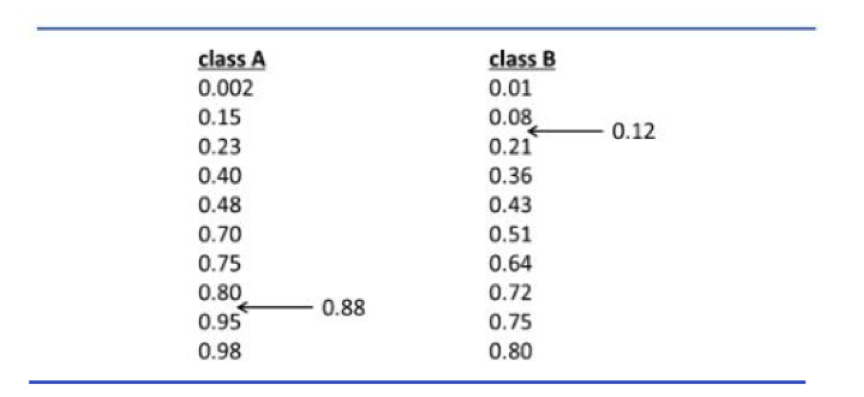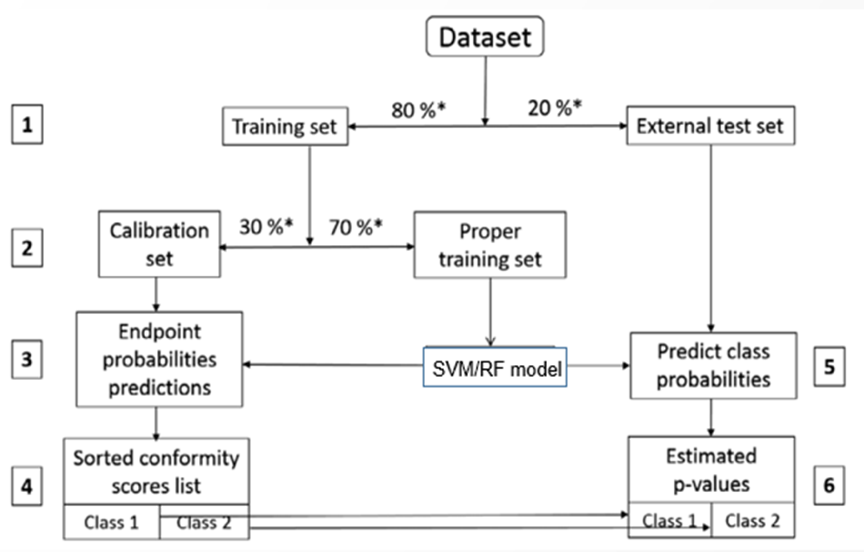QSAR模型定义域主要基于化合物的理化性质、生物信息和结构特征，有三类主要的方法：距离法，标准差法以及适形预测法。

## 距离法

$$D_T=\overline{\gamma} + Z\sigma$$

## 标准差法

$$STD(J)=\sqrt{\frac{\sum^k_{i=1}{(y_i-\overline{y})^2}}{k-1}}$$

## Conformal Prediction 适形预测法